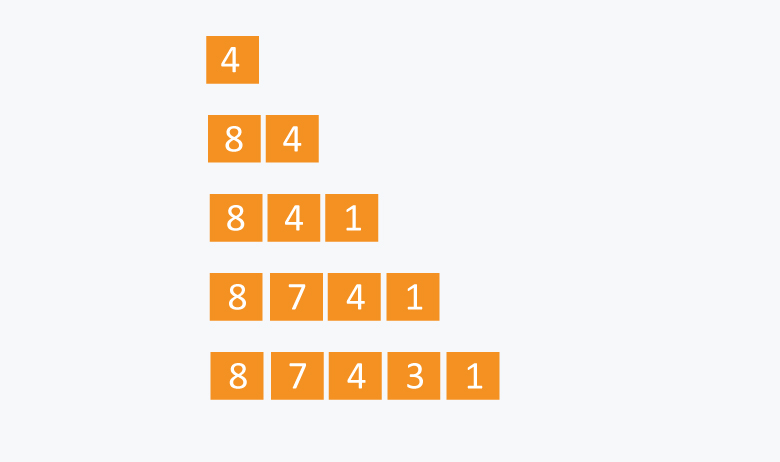×

Search anything:

# Implementing Priority Queue using Linked List

#### Algorithms Data Structures queue priority queueA queue is a FIFO data structure i.e First In First Out.
Priority queue as the name suggests should have the elements or tasks ordered with respect to priority in the queue.If two elements have the same priority than they
will be executed in the sequence they are added in the list.

The main operations for implementing a priority queue are:

1. Push operation:This function is used to insert new data into the queue based on priority.

### Pseudocode for Push operation:

• Step 1: Create a new node of structure node type.
• Step 2: Check if HEAD has lower priority. If true follow Steps 3-4 and end. Else goto Step 5.
• Step 3: NEW -> NEXT = HEAD.
• Step 4: HEAD = NEW.
• Step 5: Set TEMP to head of the list.
• Step 6: While TEMP -> NEXT != NULL and TEMP -> NEXT -> PRIORITY > PRIORITY
• Step 7: TEMP = TEMP -> NEXT.
• [END OF LOOP]
• Step 8: NEW -> NEXT = TEMP -> NEXT.
• Step 9: TEMP -> NEXT = NEW.
• Step 10: End.

Explanation:
For each push operation we start with checking head priority and proceed.Then to get to the correct priority node in the list we loop it with the 'while' condition and end it just before the correct position for our new data and we link it in the list.

``````//Push operation in CPP
void push(Node** head, int d, int p)
{
Node* temp = newNode(d, p);
{
}
else
{
while(start->next !=NULL && start->next->priority < p)
{
start = start->next;
}
temp->next = start->next;
start->next = temp;
}
}
``````

• Step 1: Set the head of the list to the next node in the list. HEAD = HEAD -> NEXT.
• Step 2: Free the node at the head of the list.
• Step 3: End.

### Explanation:

The pop operation is a simple O(1) operation as we always have a high priority element ready to be popped out.We link the head to the next priority element and free the node.

• Step 1: Return HEAD -> DATA.
• Step 2: End.

Explanation:
This is just to read the highest priority element of queue.

``````//TOP OPERATION
``````

## Example for Priority Queue Implementation:

Let’s say we have an array of 5 elements : {4, 8, 1, 7, 3} and we have to insert all the elements in the max-priority queue.

• First as the priority queue is empty, so 4 will be inserted initially.
• Now when 8 will be inserted it will moved to front as 8 is greater than 4.
• While inserting 1, as it is the current mi nimum element in the priority queue, it will remain in the back of priority queue.
• Now 7 will be inserted between 8 and 4 as 7 is smaller than 8.
• Now 3 will be inserted before 1 as it is the 2nd minimum element in the priority queue.

All the steps are represented in the diagram below:### Implementations

Following is the implementation of Priority queue for a demo :

``````#include <bits/stdc++.h>
using namespace std;
// Node
typedef struct node
{
int data;
int priority;//lower value high priority
struct node* next;
} Node;

// Function to create a new node
Node* newNode(int d, int p)
{
Node* temp = (Node*)malloc(sizeof(Node));//Dynamic Memory allocation
temp->data = d;
temp->priority = p;
temp->next = NULL;

return temp;
}

// Return the value at head
{
}
//Removes the highest priority node.
{
free(temp);//Freeing the extra memory
}

// Function to push according to priority
void push(Node** head, int d, int p)
{
Node* temp = newNode(d, p);
{
}
else
{
while (start->next != NULL && start->next >priority < p)
{
start = start->next;
}
temp->next = start->next;
start->next = temp;
}
}
//To check whether the queue is empty or not
{
}

int main()
{
// Creating a Priority Queue
// 8->7->4->3->1

{
cout << " " << top(&head);
}
return 0;
}
``````

### Complexity

• Push case time complexity: `O(n)`
• Pop case time complexity: `O(1)`
• Top case time complexity: `O(1)`

### Applications

Some applications of priority Queues:

• Priority queues are used in operating system for load balancing and interrupt handling.
• Dijkstra's shortest path algorithm implementation can be done using priority queues.
• Priority queues are used to sort heaps.
• Priority queues are used in huffman codes for data compression.